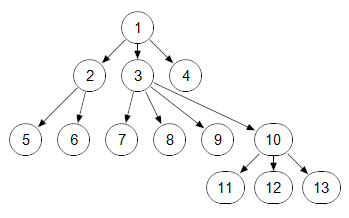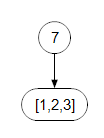# Repeated Parameter Forwarding

Repeated parameter forwarding lets you pass a list of items to a repeated function parameter. A repeated function parameter, with qualifier "..." accepts one or more parameters of the specified type. If you don't know about repeated parameters, you may want to first read Repeated parameters section of Function Parameter Qualifiers.

## Basic idea

Consider this User-Defined Function:

Function TreeNode(x; children:...)
Definition: Array(TreeNodeIdx, [x, \children])

where

Index TreeNodeIdx := ['value', 'children']

Then TreeNode(1, 2, 3, 4) creates this data structure:The repeated parameter, «children», allows you to list as many children as you'd like, without having to make use of square brackets in the syntax. Hence, we could built a multi-level tree using:

TreeNode(1, TreeNode(2, 5, 6), TreeNode(3, 7, 8, 9, TreeNode(10, 11, 12, 13)), 4)Now suppose you have some expression -- say, F() -- that computes and returns a list of "nodes", and you want to create a tree node having this set of nodes as the children, with 7 as the root. Your expression doesn't know what F() will return, or even how many children it will return, but for illustration let's pretend it returns [1, 2, 3]. Your first attempt might be

TreeNode(a, F())

This produces an error, because you cannot pass a list value to a repeated parameter; however, logically what you are actually doing here is producing the wrong tree, which would actually beThe call is providing one instance of the repeated parameter, hence one child node. The value of that child node is the list of values. What we really want is to be able to pass three instances of the «children» parameter to the function, basically

TreeNode(7, Slice(F(),1), Slice(F(),2), Slice(F(),3))

This would work if we knew that F() would return 3 values -- but Repeated parameter forwarding lets us handle this when we don't know how many items F() will to return. To forward the result of F() to the repeated parameter, we preface it with three dots

TreeNode(7, ...F())

The three dots (or ellipsis, which matches the three dots used when declaring a repeated parameter) indicate that we want each item in the computed result to be passed as one instance of the repeated parameter. When you use this syntax, the expression following the three dots must produce a list or a 1-D array.

Be aware that ... is part of the expression -- not that we've omitted something!

## Uses

### Operating over all indexes of an array

Several built-in functions accept a repeated index parameter, so that you can apply them over multiple dimensions, such as:

Sum(X, I, j, K)

Other such functions, besides Sum, include Max, Min, ArgMax, ArgMin, CondMax, CondMin, Average, Product, and Array.

Sometimes you'd like to apply these functions over every index of an array. Parameter forwarding makes it easy, e.g.

Sum(A,...IndexesOf(A))

Note that this sometimes violates the principle of array abstraction, which could limit the flexibility of your model. So you should be careful with this.

### Creating a constant array over computed dimensions

Sometimes it is useful to create a constant array over a set of indexes. You can do this using, e.g, Array(I, J, 0), to create an array on indexes I and J having the value 0 everywhere. But what if the set of indexes is computed? Here is an example

Array( ...#SetDifference(\IndexesOf(A), \IndexesOf(B)), 0)

This creates an array containing all the indexes of A that are not also indexes of B, with all values 0.

### Forwarding a repeated parameter in a user-defined function

Sometimes it's useful to wrap an existing function with a User-Defined Function that you create. For example, if an existing function does most of what you want, but doesn't treat Null values the way you like, has a bug you need to work around, or for some other reason you might create your own variation. The original function might have a bunch of parameters that you just simply want to forward to the original function. When the parameter being forwarded is a repeated parameter, you need to use the repeated parameter forwarding syntax.

The User-Defined Function AbsMax here accepts the same parameters as Max, but returns the maximum of the absolute value. It is essentially a wrapper of the Max function -- we copy the parameter declaration for Max:

Function AbsMax(X; I: ... recommended Index WarnDynamic; IgnoreNonNumbers, IgnoreNaN: optional named atomic boolean; caseInsensitive: optional atomic boolean)
Definition: Max(Abs(X),...I, IgnoreNonNumbers, IgnoreNaN, caseInsensitive)


To forward the repeated parameter, we need the ... in front of I, otherwise it would look like one parameter instance.

### Computing a set of decisions or constraints for DefineOptimization

The DefineOptimization function accepts a list of decision and constraint nodes as repeated parameters. If you decide to get fancy, you might decide to create an interface, or some logic, that computes which constraints or decisions should be active. Repeated parameter forwarding allows you to provide a computed list of decisions or constraints to the optimization declaration, e.g. DefineOptimization(decisions: ...ActiveDecisions, constraints: ...ActiveConstraints, maximize: myObjective )

Note that although the two parameters normally look for object identifiers, here the identifier ActiveDecisions is actually the variable that computes which decisions to use (as a 1-D array or list), and not one of the decision nodes. Similarly for ActiveConstraints.

## History

Introduced in Analytica 4.5.